# KSEEB Solutions for Class 6 Maths Chapter 5 Understanding Elementary Shapes Ex 5.1

Students can Download Chapter 5 Understanding Elementary Shapes Ex 5.1 Questions and Answers, Notes Pdf, KSEEB Solutions for Class 6 Maths helps you to revise the complete Karnataka State Board Syllabus and score more marks in your examinations.

## Karnataka State Syllabus Class 6 Maths Chapter 5 Understanding Elementary Shapes Ex 5.1

Question 1.
What is the disadvantage in comparing line segments by mere observation?
Solution:
By observation, we cannot be absolutely sure about the judgement When we compare two lines segments of almost same lengths, we cannot be sure about the line segment of greater length therefore, it is not an appropriate method to compare line segment having a slight difference!’ between their lengths. This is the disadvantages in comparing line segments by mere observation.

Question 2.
Why is it better to use a divider than a rule, While measuring the length of a line segment?
Solution:
It is better to use a divider than a ruler because While using a ruler, positioning error may occur due to the incorrect positioning of the eye.Question 3.
Draw any line segment, say $$\overline{\mathbf{A B}}$$. Take any point C lying in between A and B Measure the lengths ofAB, BC and Ac. Is AB = AC + CB?
[Note: If A, B, C are any three points on a line such that AC + CB = AB, then we can be sure that C lies between A and B]
Solution:
It is given that point C is lying somewhere in between A and B Therefore all these points are lying on the same line segment $$\overline{\mathbf{A B}}$$. Hence for every situation in Which point C is lying in between A and B, it may be said that AB = AC +CB
For example:
$$\overline{\mathbf{A B}}$$ is a line segment of 6 Cm and C is a point between A and B, Such that it is 2 cm away from point B. We can find that the measure of line segment $$\overline{\mathbf{A C}}$$ comes to 4 cm Hence, relation AB = AC + CB is verified

Question 4.
If A, B, C are three points on a line such that AB = 5 cm, BC = 3 cm and AC = 8 cm, Which one of them lies between the other two?
Solution:
Given that AB = 5 cm
BC = 3 cm
AC = 8 cm
it can be observed that AC = AB + BC
Clearly, point B is lying between A and C.Question 5.
Verify, Whether D is the mid point of $$\overline{\mathbf{A G}}$$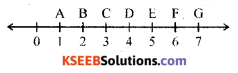Solution:
From the given figure. it can be observed that
$$\overline{\mathbf{A D}}$$ = 4 – 1 = 3 units
$$\overline{\mathbf{D G}}$$ = 7 – 4 = 3 units
$$\overline{\mathbf{A G}}$$ = 7 – 1 = 6 units
Clearly 0 is the mid – point of AG

Question 6.
If B is the mid point of $$\overline{\mathbf{A C}}$$ and C is the mid point of $$\overline{\mathbf{B D}}$$, Where A, B, C, D lie on a straight line, say Why AB = CD?
Solution: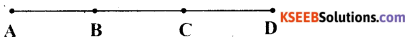Since B is the mid – point ofAC
AB = BC ……. (1)
Since C is the mid point of BD
BC = CD …… (2)
From equation (1) and (2), we may find that AB = CD

Question 7.
Draw five triangles and measure their sides, Check in each case, if the sum of the lengths of any two sides is always less than the third side.
Solution:
i)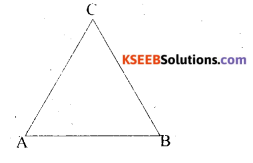In above ∆ le AB = 3 cm
BC = 3 cm
CA = 3 cm
So AB + BC > CA
AB + CA > BC
BC + CA > ABii)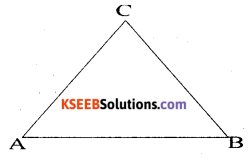In above ∆ le AB = 6 cm
BC = 5 cm
BC = 5.3 cm
So AB + BC > CA
AB + CA > BC
AB + CA > AB

iii)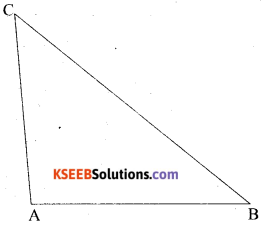In above ∆ ABC AB = 4 cm
AC = 3 cm
BC = 5 cm
So AB + AC > BC
AB + BC > AC
BC + CA > AB

iv)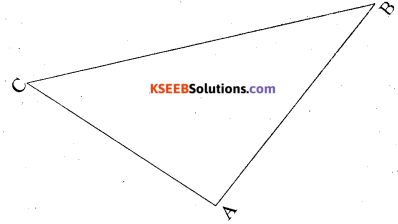In above ∆ ABC AB = 7 cm
BC = 7 cm
AC = 4 cm
So AB + BC > BA
AB + BC > AC
BC + CA > ABv)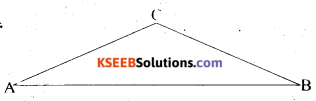In above ∆ ABC AB = 10 cm
AC = 4 cm
BC = 5 cm
So AB + BC > AC
AB + AC > BC
BC + CA > AB
AC + CB > AB
In the above following any two sides always less than the third side.